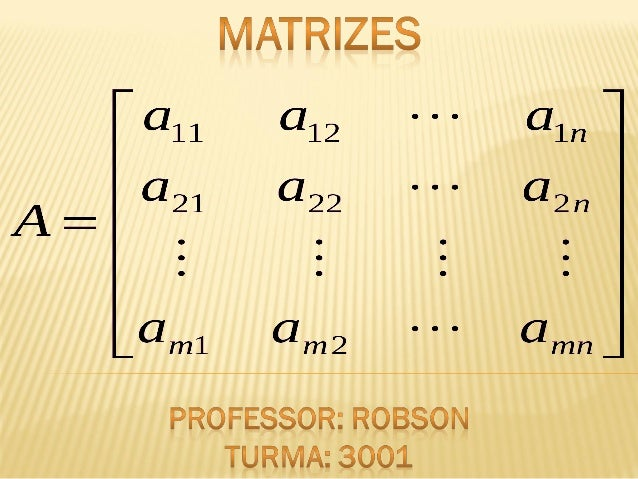Successfully reported this slideshow.
We use your LinkedIn profile and activity data to personalize ads and to show you more relevant ads. You can change your ad preferences anytime.Upcoming SlideShare
Loading in …5
×

# Matrizes - Completo com exercícios

60,055 views

Published on

Aqui temos a introdução do assunto matrizes e exercícios para vocês tentarem resolver, qualquer dúvida é só comentar aqui que eu explico o exercício. Obrigada!

Published in: Education
• Full Name
Comment goes here.

Are you sure you want to Yes No
Your message goes here• Be the first to comment

### Matrizes - Completo com exercícios

1. 1.  Origem da matriz:  O primeiro vestígio de matrizes foi escrito durante a dinastia Han entre  200 a.C e 300 a.C no texto texto “Nove Capítulos da Arte Matemática”. Mas o 1º a usar o termo “matriz” foi Sylvester em 1850 que ao voltar a Inglaterra conheceu Cayley e compartilharam seus interesses na matemática. Cayley percebeu rapidamente o significado do conceito de matriz e por volta de 1853, Cayley havia publicado uma nota apresentando pela primeira vez a inversa de uma matriz.
2. 2.  O uso das matrizes no dia-a-dia: • Imagens de internet (GIF, JPEG)
3. 3. • Planilhas eletrônicas (Excel)
4. 4.  Uma matriz pode ser representada de três formas: 1 2 3 4 5 6 1 2 3 4 5 6 1 2 3 4 5 6 Colchetes Parênteses Barra dupla
5. 5. Elemento Coluna Coluna Coluna Linha Linha
6. 6.  Vamos ver algumas definições úteis: Matriz A ( m linha e n colunas ) Elemento qualquer que está na linha i e na coluna j
7. 7.  Uma matriz pode ser descrita também através de uma lei de formação. Exemplo: A = (a ) onde a = i + j: ij 2x3 ij A = (a )ij 2x3 a = i + j ij A = =
8. 8.  Matriz linha: Só tem uma linha. Exemplo:
9. 9.  Exercício - Matriz linha:  a) Escreva a matriz linha do tipo 1x4 tal que aij = 2i + 3j.
10. 10.  Matriz coluna: Só tem uma coluna  Exemplo: A =
11. 11.  Matriz quadrada: m = n  Exemplo 1: Exemplo 2:
12. 12.  Exercícios – Matriz quadrada  (PUCC–SP–Adaptada) Seja a matriz A = ( aij ) 2 x 2, em que aij = i + j, se i = j e i – j, se i ≠ j.
13. 13.  Matriz triangular inferior: Matriz em que os elementos acima da diagonal são iguais a zero.  Exemplo 1: Exemplo 2:
14. 14.  Matriz triangular superior: Matriz em que os elementos abaixo da diagonal principal são iguais a zero.  Exemplo 1: Exemplo 2: A =
15. 15.  Matriz diagonal Exemplo 1: Exemplo 2:
16. 16. Exercício – Matriz diagonal Escreva a matriz diagonal de 4ª ordem tal que os elementos diferentes de zero satisfaçam à seguinte condição aij = i - 3j.
17. 17.  Matriz identidade: os elementos que pertencem à diagonal principal são sempre iguais a 1 e os outros elementos que não pertencem à diagonal principal são iguais a zero.   Exemplo: Matriz de ordem 2: Matriz de ordem 3:
18. 18.  Matriz nula: é qualquer matriz onde todos os elementos são 0.  A =
19. 19.  Se A = B, então: • a= • b= • c= • d= A = 1 2 3 4 B = a c b d
20. 20.  Exercício: Determine x e y para que as matrizes A e B sejam iguais:
21. 21.  Se duas matrizes possuem a mesma ordem, basta somarmos os elementos correspondentes. Exemplo: A = 1 2 3 4 5 6 B = 2 5 4 1 2 9 A + B = =
22. 22.  Exercício:  Determine a matriz C, resultado da soma da matriz A e B:  A = 2 0 -4 B = 2 3 2 10 7 1 0 4 7 C = C =
23. 23.  Exemplo: 2 . 1 2 3 4 =
24. 24.  Considerando a matriz: -2 3 4 -5 , determine: a) 4 . ( A + B) A= E B= 1 0 2 1
25. 25.  Exercício: Determine a matriz oposta de e depois determine (–A + A):
26. 26.  Para calcular A-B as matrizes devem ser da mesma ordem.  Exemplo: Dada a matriz A =  e a matriz B = , se efetuamos a subtração dessas matrizes, temos:
27. 27.  Para que seja possível: colunas da 1ª matriz deve ser igual ao nº de linhas da segunda. Exemplo: B = 2 1 3 2 4 5 A = 1 2 3 3 1 1
28. 28.  Exercício: Seja A= 1 4 e B= 1 : 2 5 3 6 2 a) Existe o produto AB? Justifique: b) Existe o produto BA? Justifique: c) Calcule o produto AB:
29. 29.  Exemplo 1:  Exemplo 2: B =
30. 30.  Exercício: a) Determine a matriz do tipo 3x1 tal que aij = i.3 + 3j. b) Determine a matriz transposta da obtida no item A.
31. 31.  É quando uma matriz e sua transposta são iguais. Exemplo: Dada a matriz A = , sua transposta será?  Toda matriz identidade é simétrica.
32. 32.  A . A = In e A . A = In  A= 5 1 4 1 -1 -1